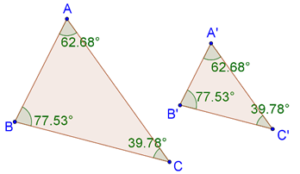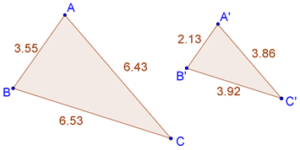# Similarity in Triangles

Similarity in Triangles
Go back to  'Triangles'

## When are two triangles said to be similar?

Two triangles are said to be similar if their:

• corresponding angles are equal

• corresponding sides are in the same ratio

## Solved Example:

Example 1: Consider the two similar triangles as shown below:Because they are similar, their corresponding angles are the same. This means that:

\begin{align} \angle A &= \angle A' \\ \angle B &= \angle B' \\ \angle C &= \angle C' \\ \end{align}

Also, their corresponding sides will be in the same ratio. This means that:

$\frac{{AB}}{{A'B'}} = \frac{{BC}}{{B'C'}} = \frac{{AC}}{{A'C'}}$

Example 2: Consider the following example of two similar triangles:Note that the corresponding sides are in the same proportion:

$\frac{{3.55}}{{2.13}} = \frac{{6.53}}{{3.92}} = \frac{{6.43}}{{3.86}} \approx 1.67$

The numerical values of the ratios, as shown above, might not be exactly the same (due to finite errors in length measurement), but in principle, these ratios must be exactly the same.Think: Are all isosceles triangles similar to each other?

Download Similar Triangles Worksheets
Similar Triangles
grade 9 | Questions Set 2
Similar Triangles
grade 9 | Answers Set 2
Similar Triangles
grade 9 | Questions Set 1
Similar Triangles
grade 9 | Answers Set 1

More Important Topics
Numbers
Algebra
Geometry
Measurement
Money
Data
Trigonometry
Calculus